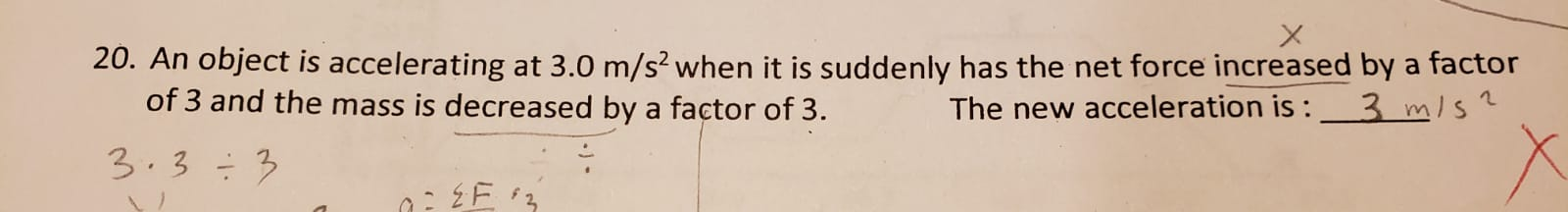# 20. An object is accelerating at 3.0 m/s? when it is suddenly has the net force increased by a factorof 3 and the mass is decreased by a factor of 3.3 m/s?The new acceleration is :3.3÷3

Question
9 viewshelp_outlineImage Transcriptionclose20. An object is accelerating at 3.0 m/s? when it is suddenly has the net force increased by a factor of 3 and the mass is decreased by a factor of 3. 3 m/s? The new acceleration is : 3.3÷3 fullscreen
check_circle

Step 1

Given:

Original acceleration = 3 m/s2

New force = 3F

New mass = m/3

Step 2

Original acceleration can also be given as:

Step 3

New acceleration can al...

### Want to see the full answer?

See Solution

#### Want to see this answer and more?

Solutions are written by subject experts who are available 24/7. Questions are typically answered within 1 hour.*

See Solution
*Response times may vary by subject and question.
Tagged in

### Physics